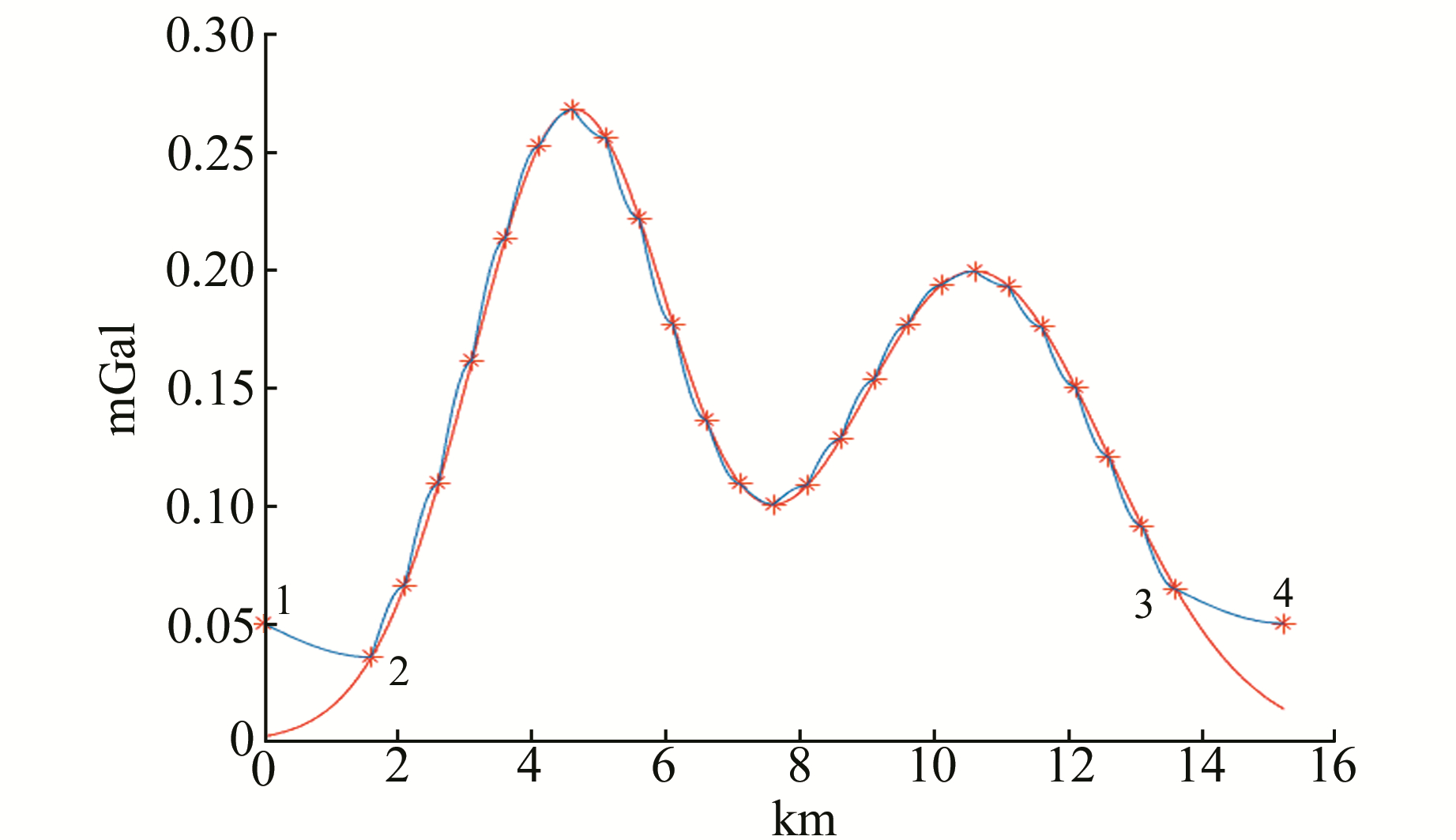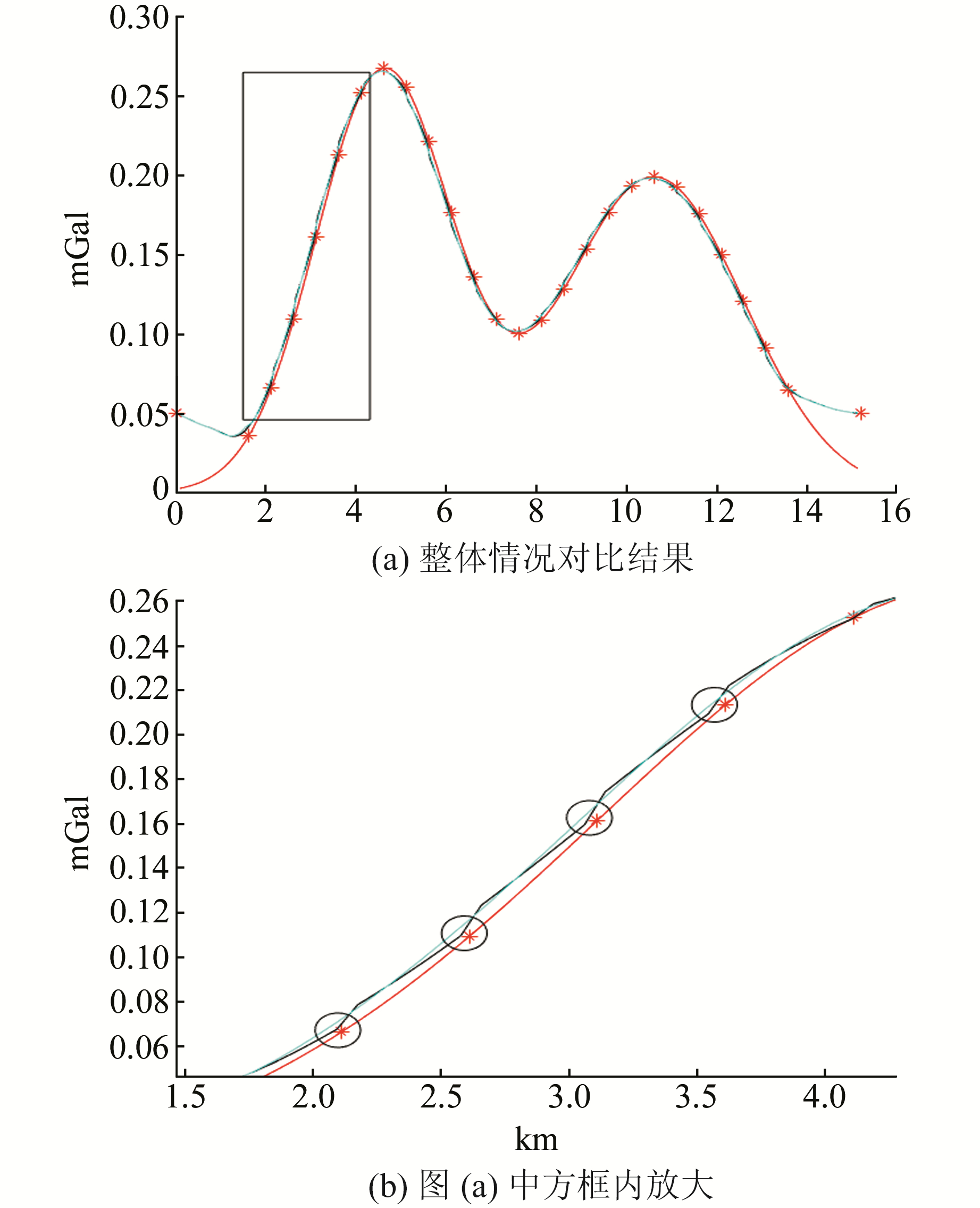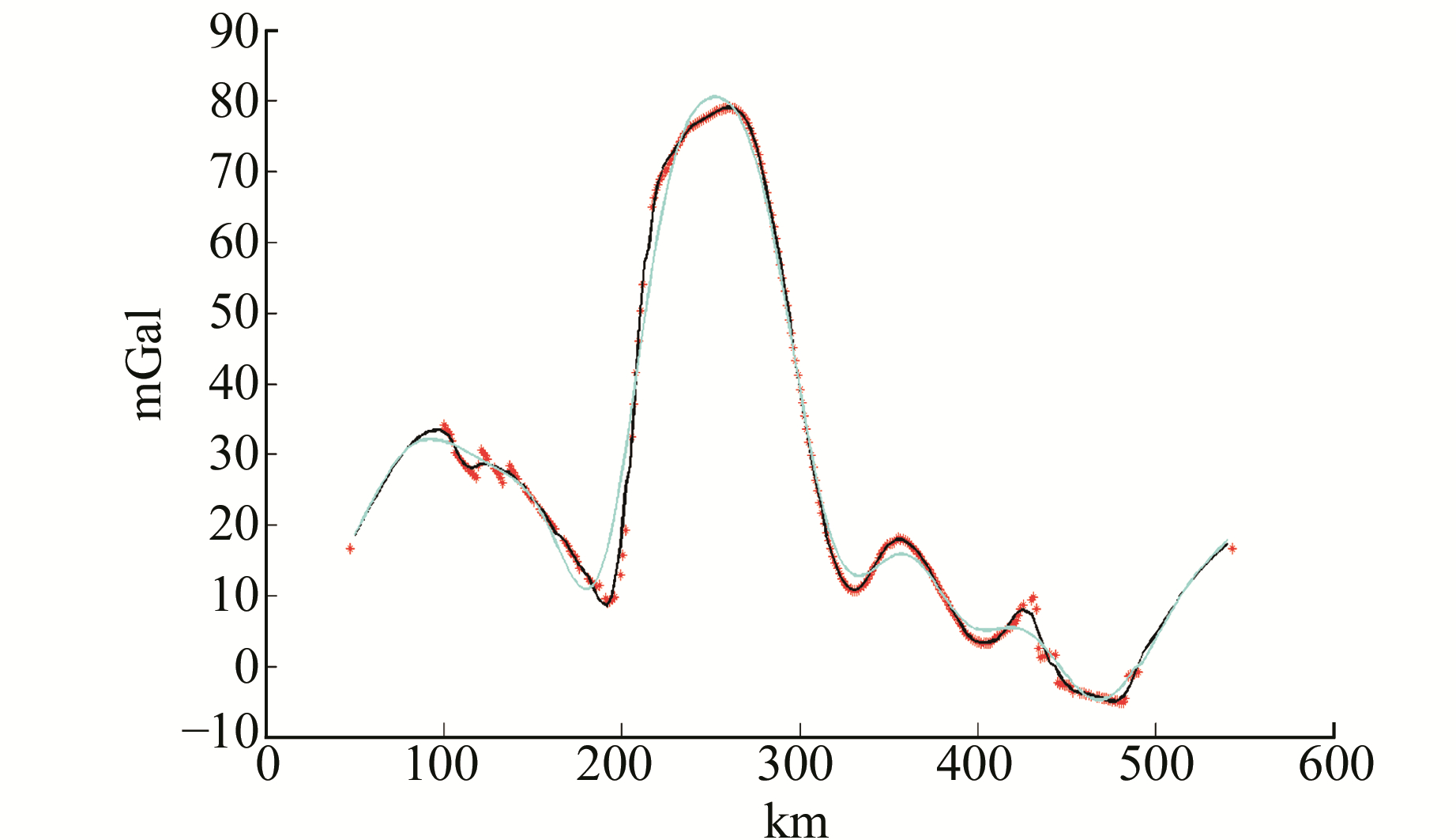﻿ 改进的最小曲率迭代法在重力剖面数据插值中的应用文章快速检索 高级检索
 大地测量与地球动力学2019, Vol. 39Issue (8): 810-812  DOI: 10.14075/j.jgg.2019.08.008### 引用本文ZHU Wenwu, PENG Junhuan, GAO Yanlong, et al. Improved Minimum Curvature Iteration Algorithm for Intrapolation and Extrapolation of Gravity Profile Data[J]. Journal of Geodesy and Geodynamics, 2019, 39(8): 810-812.### Foundation support

National Key Research and Development Program of China, No.2018YFC1503606;Seismic Regime Tracking Project of CEA, No.2019010205;Special Project of Basic Work of Science and Technology, Ministry of Science and Technology, No.2015FY210400.

### 第一作者简介

ZHU Wenwu, PhD candidate, majors in data processing in gravity and leveling, E-mail:fmccea@163.com.

### 文章历史

1. 中国地质大学(北京)土地科学技术学院，北京市学院路29号，100083;
2. 中国地震局第一监测中心，天津市耐火路7号，300180

1 算法原理

 $\begin{array}{c} \mu \left( i \right) = - {\rm{ }}\frac{1}{6}{\rm{ }}\{ \mu \left( {i + 2} \right) + \mu \left( {i - 2} \right) - 4[\mu \left( {i + 1} \right) + \\ \mu \left( {i - 1} \right)]\} , i = 1, 2, \ldots , n \end{array}$ (1)

 $\begin{array}{c} {\mu ^{(k)}}\left( i \right) = - {\rm{ }}\frac{1}{6}{\rm{ }}\{ {\mu ^{(k - 1)}}\left( {i + 2} \right) + {\mu ^{(k)}}\left( {i - 2} \right) - \\ 4[{\mu ^{(k - 1)}}\left( {i + 1} \right) + {\mu ^{(k)}}\left( {i - 1} \right)]\} , i = 1, 2, \ldots , n \end{array}$ (2)
2 算例 2.1 理论算例

 $f\left( x \right) = {\rm{ }}\frac{1}{{\sqrt {2{\rm{ \mathsf{ π} }} {\delta _1}} }}{\rm{e}}^{{\frac{{ - \left( {x - \mu } \right)2}}{{2{\delta_1 ^2}}}}} + {\rm{ }}\frac{1}{{\sqrt {2{\rm{ \mathsf{ π} }} {\delta _2}} }}{{\rm{e}}^{\frac{{ - {{(x + \mu )}^2}}}{{2\delta _2^2}}}}$ (3)红线：原函数曲线；蓝线：余弦变换；红色*：约束点，2个端点为外推的端点值 图 1 原函数曲线与余弦变换结果 Fig. 1 Comparison of real value and calculation results with cosin transformation红线：原函数曲线；红色*：约束点，2个端点为外推的端点值（由约束点外侧2个点求平均获得）；黑线：改进的最小曲率计算结果；青线：传统的最小曲率计算结果 图 2 改进前后最小曲率迭代方法解算对比结果 Fig. 2 Comparison of the minimum curvature calculation before and after modification

 $v = {\rm{ }}\sqrt {\frac{{{{\left( {{f^\prime } - f} \right)}^2}}}{N}}$ (4)表 1 改进前后的差异性统计计算精度 Tab. 1 Statistical calculation accuracy of difference before and after improvement
2.2 实际算例红色*：约束点; 黑线：改进的最小曲率计算结果；青线：传统的最小曲率计算结果 图 3 改进方法与传统方法插值和外推结果 Fig. 3 Comparison of the minimum curvature calculation before and after improvement
3 结语

  申重阳, 杨光亮, 谈洪波, 等. 维西-贵阳剖面重力异常与地壳密度结构特征[J]. 地球物理学报, 2015, 58(11): 3 952-3 964 (Shen Chongyang, Yang Guangliang, Tan Hongbo, et al. Gravity Anomalies and Crustal Density Stucture Characteristics of Profile[J]. Chinese Journal of Geophysics, 2015, 58(11): 3 952-3 964) (0)  徐东卓, 朱传宝, 孟宪纲, 等. 山西中北部地区地壳垂直形变时空演化特征及与强震的关系[J]. 地震研究, 2018, 41(3): 446-450 (Xu Dongzhuo, Zhu Chuanbao, Meng Xiangang, et al. Temporal and Spatial Evolution Characteristics of Crust Vertical Deformation and Its Relationship with Strong Earthquakes in Central and Northern Shanxi[J]. Journal of Seismological Research, 2018, 41(3): 446-450 DOI:10.3969/j.issn.1000-0666.2018.03.014) (0)  王同庆, 陈石, 梁伟锋, 等. 2016年门源MS6.4地震前的区域重力场变化与定量参数分析[J]. 地震地质, 2018, 40(2): 349-360 (Wang Tongqing, Chen Shi, Liang Weifeng, et al. The Variation of Regional Gravity Field and Quantitative Parameter Analysis before 2016 Menyuan MS6.4 Earthquake[J]. Seismology and Geology, 2018, 40(2): 349-360 DOI:10.3969/j.issn.0253-4967.2018.02.005) (0)  孟庆筱, 党学会. GPS约束下九寨沟地区断裂带现今运动速率的非连续接触模拟研究[J]. 地震研究, 2018, 41(3): 390-397 (Meng Qingxiao, Dang Xuehui. Research on Crustal Deformation in the Jiuzhaigou Area under the Constraints of GPS Results by Discontinuous Contact Model[J]. Journal of Seismological Research, 2018, 41(3): 390-397 DOI:10.3969/j.issn.1000-0666.2018.03.007) (0)  骆遥, 吴美平. 位场向下延拓的最小曲率方法[J]. 地球物理学报, 2016, 59(1): 240-251 (Luo Yao, Wu Meiping. Minimum Curvature Method for Downward Continuation of Potential Field Data[J]. Chinese Journal of Geophysics, 2016, 59(1): 240-251) (0)  王万银, 邱之云, 刘金兰, 等. 位场数据处理中的最小曲率扩边和补空方法研究[J]. 地球物理学进展, 2009, 24(4): 1 327-1 338 (Wang Wanyin, Qiu Zhiyun, Liu Jinlan, et al. The Research to the Extending Edge and Interpolation on the Minimum Curvature Method in Potential Field Data Processing[J]. Progress in Geophysics, 2009, 24(4): 1 327-1 338) (0)  王万银, 邱之云. 一种稳定的位场数据最小曲率网格化方法研究[J]. 地球物理学进展, 2011, 26(6): 2 003-2 010 (Wang Wanyin, Qiu Zhiyun. The Research to a Stable Minimum Curvature Gridding Method in Potential Data Processing[J]. Progress in Geophysics, 2011, 26(6): 2 003-2 010) (0)  王万银. 位场数据处理中的最小曲率方法及Fortran语言程序设计[M]. 北京: 地质出版社, 2010 (Wang Wanyin. The Minimum Curvature Method in Geophysical Data Processing and Fortran Programme Design[M]. Beijing: Geological Publishing House, 2010) (0)  Love A E H. The Mathematical Theory of Elasticity[M]. Cambridge: Cambridge University Press, 1926 (0)
Improved Minimum Curvature Iteration Algorithm for Intrapolation and Extrapolation of Gravity Profile Data
ZHU Wenwu1,2PENG Junhuan1     GAO Yanlong2     LI Fangzhou2     JIA Yue2
1. School of Land Science and Technology, China University of Geosciences, 29 Xueyuan Road, Beijing 100083, China;
2. The First Curst Monitoring and Application Center, CEA, 7 Naihuo Road, Tianjin 300180, China
Abstract: We discover a new minimum curvature algorithm, based on the idea that the constraints are treated as unknown sites. The improved method avoids complicated reduction, without the need to consider the relationship between constraint points and unknown points when the constraint points exist around the unknown points. Furthermore, precision is improved.
Key words: gravity profile; minimum curvature; cosine transformation; difference equation